Transition from the general equation of the line to the canonical equations

Consider the transition from the general equation of the straight line (10) to the canonical equations (11).

This transition is carried out according to ALGORITHM 1

 ALGORITHM 1 Transition from the general equation of the line to the canonical equations Given: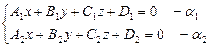Bring the canonical form to the general equation of the line. Solution. We will carry out a schematic drawing of the general equation of the line (Fig. 18)Fig. 18 1 Find the coordinates of the guide vector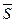. Since the line l lies in the plane α 1 , the vectoralso lies in the plane α 1 , then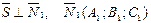Is the normal vector of the plane α 1 . Similarly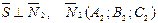We have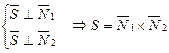then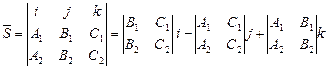2 Find the point M 0 through which the line passes. For the point M 0 take the point of intersection of a straight line with one of the coordinate planes. Let M 0 = l∩ХОУ, then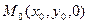, substitute the coordinates of the point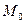in equation (9), we obtain the system of equations: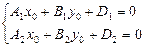We solve the resulting system, find the coordinates of the point. 3 We compose the equation of the line. Substitute the coordinates of the pointand vectorinto the canonical equations of the line (10), we obtain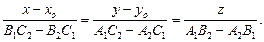They say that to find the point through which the line passes, one of the variables in the general equation of the line must be equal to zero and the resulting system of equations should be solved.

Problem 16 Reduce the canonical form of the general equation of the line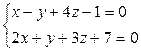.

Decision

Find the directing vector of the line. Since it should be perpendicular to normal vectorsand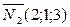given planes then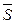can take a vector product of vectors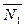and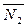:In this way,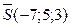As a point, through which the line passes, we can take the point of its intersection with any of the coordinate planes, for example, with the XOY plane, sincethen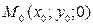-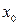andthis point is determined from the system of equations of given planes, if we put in them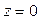: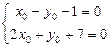Solving this system, we find: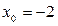,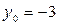, i.e.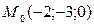Substitute the found coordinates of the point M 0 and the directing vector S into equation (2), we obtain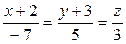.

Answer:Do it yourself

Problem 16.1. Reduce the canonical form to the general equation of the line: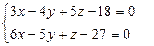Answer: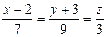.

; Date Added: 2015-06-28 ; ; Views: 30161 ; Does published material infringe copyright? | | Personal Data Protection | ORDER JOB

Did not find what you were looking for? Use the search:

Best sayings: A student is a person who constantly puts off inevitability ... 10346 -| 7270 -or read everything ...